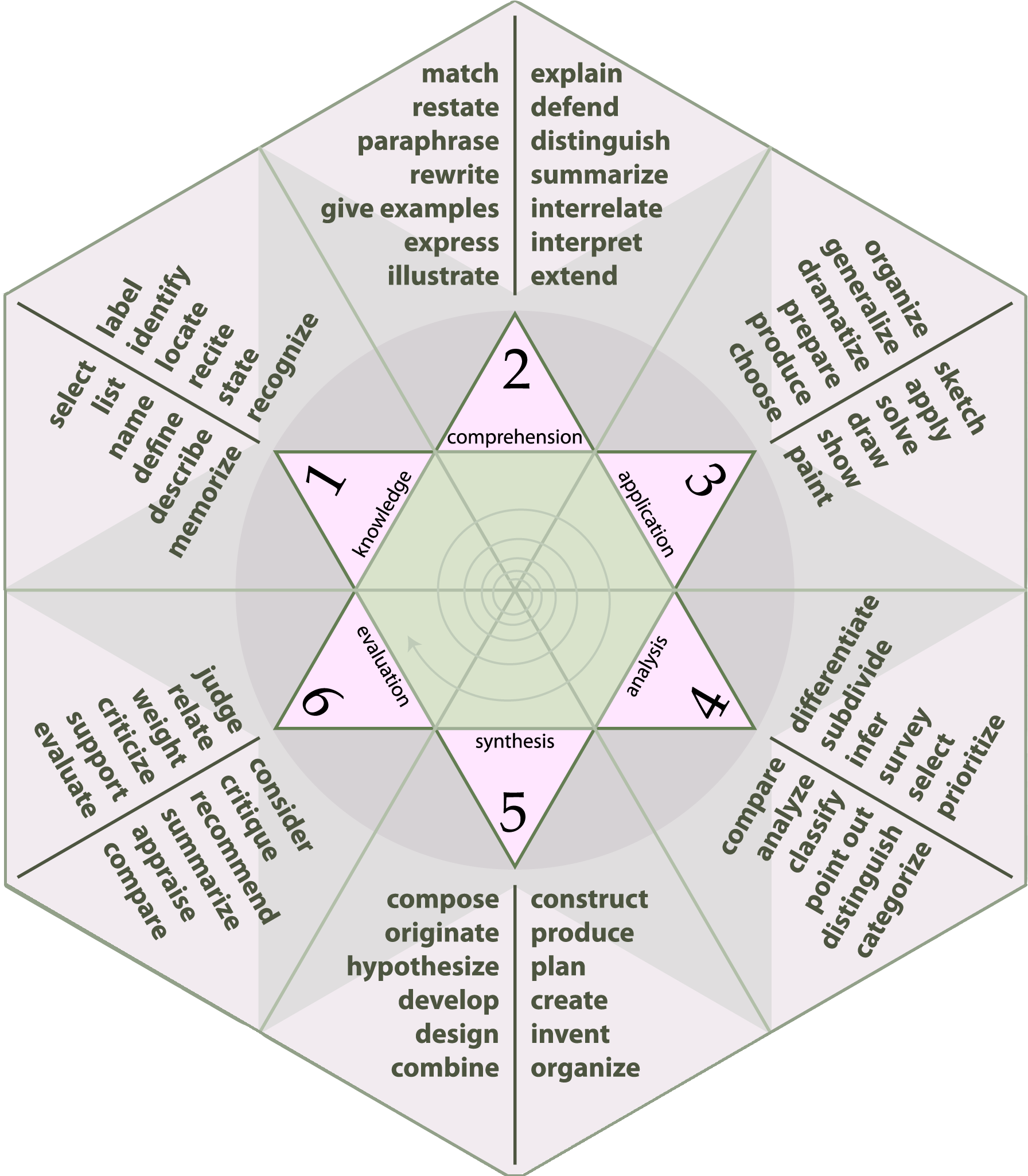## Computational Tools Objectives

Course objectives are key skills, processes, and higher-level problem solving abilities that students demonstrate in the computational course. The course is designed for all level's of Bloom's taxonomy through basic information recall in programming exercises (level 1) to higher level creative processes (level 6) in the course project.• Students will demonstrate an understanding of fundamental mathematics (including calculus, linear and nonlinear simultaneous equation solving, etc.) by using computer tools to solve problems.
• Students will be able to use a spreadsheet package to perform engineering calculations which include some of the following: economic analysis, processing and analysis of data, graphical analysis and presentation, etc.
• Students will be able to solve numerical and symbolic problems with use of advanced math software (e.g. Python Scipy).
• Students will be able to write program structures, and understand when programming is most appropriate.
• Students will be able to convert problem solving strategies to procedural algorithms.
• Students will be able to make order of magnitude estimates, assess reasonableness of solutions, and select appropriate levels of solution sophistication.
• Students will demonstrate an ability to solve engineering problems.